# Addressing the Test Challenges of MIMO Communications Systems

## Overview

This document describes the fundamental architecture of a multiple input, multiple output (MIMO) communications system. In addition, it explains the challenges of synchronization in MIMO systems and provides a test architecture that addresses this need.

## Contents

### Introduction

This tutorial is part of the National Instruments Signal Generator Fundamentals Series, which covers basic concepts about the architecture, features, or strategies for baseband IQ signal generation.

## Challenges of Wireless Communications

With each stage in their evolution, wireless communications systems are able to achieve higher throughput than before.

These systems are getting closer and closer to the theoretical maximum throughput specified by Shannon’s Equation, which is a function of channel bandwidth and signal-to-noise ratio (SNR). In fact, recent innovations have enabled wireless communications systems to achieve increasingly higher throughput with better spectral efficiency. As an example, the use of orthogonal frequency division multiplexing (OFDM) combined with higher-order modulation schemes (64-QAM) in the IEEE 802.11g standard greatly improved the throughput over the previous IEEE 802.11b standard. As the prevalence of wireless communications continues to grow, the pressure to find innovative ways to more effectively use channel bandwidth is increasing. One of the most recent innovations is the development of MIMO antennae systems to improve the SNR and increase throughput. This communications technique will be implemented in IEEE 802.11n. However, for test engineers, its complexity creates significant test challenges.

This document explores how MIMO increases channel capacity and how the fundamental characteristics of a MIMO system can create significant test challenges. It also describes how to implement hybrid PXI and traditional instrument systems to provide an effective test architecture for MIMO systems.

### Mechanisms to Maximize Spectral Efficiency

Wireless communications channels are subject to fundamental limitations in capacity the can be specified by two main factors: bandwidth and SNR. In fact,

Shannon’s equation for channel capacity reflects these relationships and is shown below: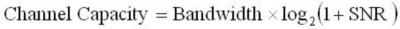Figure 1. Shannon's Equation for Theoretical Channel Capacity 

According to this equation, the capacity increases linearly with respect to an increase in bandwidth, and is logarithmically related to changes in SNR. Bandwidth is addressed in a later section, but the effect of SNR on throughput is discussed below.

### Modulation Type versus SNR

The SNR of a digital communication channel affects the throughput of the system by limiting the modulation scheme that can be used. As one might expect, more complex, higher-order modulation schemes use a greater number of symbols and can, therefore, implement more bits per symbol. For example, using a 4-QAM or 4-PSK modulation scheme ensures that a communications system is able to send two digital bits per symbol period. However, 256-QAM is able to sustain 8 bits per symbol period. Thus, simply increasing the complexity of the modulation scheme you use increases the system throughput without affecting the channel bandwidth. However, modulation schemes of higher order can be used only in communications channels with a high SNR. This is necessary because subtle changes in the phase and amplitude of the signal cannot be accurately determined in noisy channels with low SNR. In fact, as SNR decreases, the implementation of a high-order modulation scheme is not possible.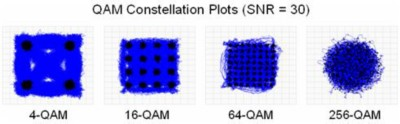Figure 2. Constellation Plots for Modulation Schemes of Increasing Order

As Figure 2 shows, individual symbols of a communications channel become difficult to distinguish from one another as the order (or M-ary) of the modulation scheme increases.  Figure 2 shows the effect of channel noise on a variety of QAM schemes, each with an energy per bit of 30.  Observe that at 256-QAM, the channel noise is too significant to enable the receiver to achieve carrier lock with the signal.  In this case, the receiver is unable to estimate the phase or frequency of the baseband signal and all transitions are indeterminable.

### Symbol Rate versus Bandwidth

The second factor of Shannon’s equation that limits system throughput is the channel bandwidth. Figure 3 shows that the bandwidth of a channel is a linear function of its symbol rate. Thus, increasing the symbol rate of the channel increases throughput at the expense of requiring more bandwidth.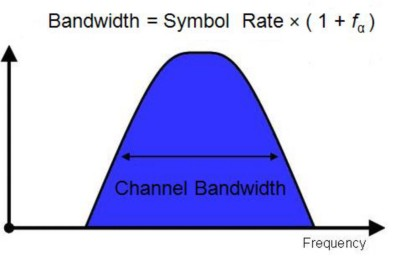Figure 3. Bandwidth versus Symbol Rate

Figure 3 shows that increasing the symbol rate of a communications channel does not improve its efficiency. Instead, it simply increases the occupied bandwidth. Because the available frequency spectrum is finite, channel bandwidth is typically limited, as illustrated by Figure 4.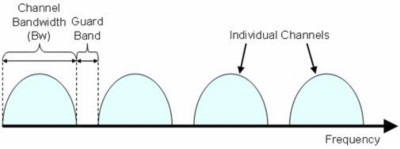Figure 4. Allocations of the Frequency Spectrum

The width of a channel determines the number of channels that can fit into a given spectrum (wider channels mean fewer channels can fit). Thus, higher symbol rates do not provide a communications system with any gain in efficiency. Moreover, while Shannon’s equation for channel capacity offers a rough idea of the theoretical limits of a communications system, this model assumes that a given channel makes optimal use of the frequency spectrum.

## Increasing Channel Efficiency with OFDM

Orthogonal frequency division multiplexing (OFDM) systems provide a substantial increase in channel throughput with improvements in spectrum efficiency. Thus, OFDM is used today in current protocols such as IEEE 802.11g (Wi-Fi) and IEEE 802.16 (WiMAX). With OFDM systems, it is possible to increase throughput in a given channel without increasing channel bandwidth or the order of the modulation scheme. This is done using digital signal processing methods that enable a single channel to be created out of a series of orthogonal subcarriers.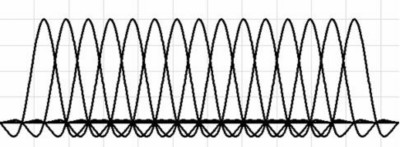Figure 5. Frequency Domain of an Overlapping OFDM Subcarrier

As Figure 5 illustrates, subcarriers are orthogonal to one another such that the maximum power of each subcarrier corresponds with the minimum power (zero-crossing point) of the adjacent subcarrier. In a typical system, the bit stream for a channel is multiplexed across various subcarriers. These subcarriers are processed with an inverse Fourier transform (IFT) and combined into a single stream. As a result, multiple streams can be transmitted in parallel while preserving the relative phase and frequency relationship between them.

In a typical system, some of these subcarriers can be used as a guard band to prevent adjacent channel interference. As an example, the frequency domain in Figure 6 shows a typical spectral allocation of a WiMAX channel.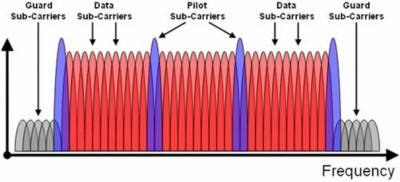Figure 6. Frequency Domain Utilization of WiMAX

Figure 6 shows that nearly twice as many subcarriers can be used in a given bandwidth because the subcarriers are able to overlap.

OFDM channel implementations have been able to increase channel throughput mainly because of gains in spectrum efficiency. By overlapping each subcarrier, engineers can effectively double the number that can be fit into a given bandwidth. In addition, OFDM systems provide two related benefits in wireless systems such as reduced multipath distortion and low intersymbol interference (ISI). In fact, because OFDM channels use relatively narrowband subcarriers, they can sample each subcarrier at low symbol rates without sacrificing throughput. Thus, these systems experience only minimal ISI, which is typically caused by multipath signal propagation of systems with higher symbol rates. In fact, many protocols that use OFDM implement a small fade interval to allow for multipath signal components to fade before the next symbol.

## Calculating Theoretical Channel Throughput

As discussed earlier, the maximum throughput for a given channel is limited by factors such as SNR, bandwidth, and spectrum efficiency (OFDM). This can be stated mathematically with the Claude Elwood Shannon's equation for theoretical throughput of a communications channel, shown in Figure 7: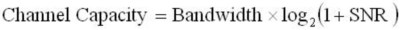Figure 7. Equation for Theoretical SISO Channel Capacity 

However, the equation in Figure 7 assumes a communications channel with one transmitter antenna and one receiver antenna. Thus, Shannon's equation can be expanded to account for systems with MIMO antennae. In MIMO systems, using multiple antennae can increase the effective SNR of the system. Moreover, the mathematical representation of the throughput of a perfectly ideal communications channel can be shown with the following equation: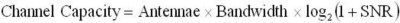Figure 8. Equation Theoretical MIMO Channel Capacity 

As Figure 8 illustrates, the channel throughput of MIMO systems can be greatly enhanced by increasing the number of transmit or receive antennae. Thus, MIMO systems are a significant innovation of wireless communications because they enable an increase in throughput of a communications channel without increasing the occupied bandwidth or order of modulation scheme. For this reason, evolving Wi-Fi standards such as IEEE 802.11n utilize the MIMO approach with OFDM to achieve significant channel throughputs. In fact, the specification aims to achieve data throughputs of more than 600 Mb/s for a 40 MHz bandwidth. This is a significant improvement over the 54 Mb/s throughput in a 20 MHz channel for IEEE 802.11g.

## MIMO Theory of Operation

The basic premise of MIMO antennae systems is that the effective SNR of the system can be increased by transmitting unique bit streams with multiple transmit antennae in the same physical channel. This is referred to as spatial multiplexing. By multiplexing the message bit stream across several channels, engineers can increase system throughput linearly according to the number of transmitters used. Note that OFDM uses a similar approach in that it also multiplexes a bit stream across each of its subcarriers. However, in OFDM systems, the symbol rate of each of the subcarriers is proportional according to the number of subcarriers in a given channel. With MIMO systems, the bit stream is multiplexed to multiple transmitters without changing the symbol rate of each independent transmitter. Thus, by adding more transmitters, engineers increase the throughput of the system without affecting the channel bandwidth.

### Spatial Multiplexing

Spatial multiplexing is the process by which a single data stream is multiplexed into individual data streams.  This is a significant departure from the traditional approach of using more of the limited bandwidth resources to increase the data rate. A block diagram of spatial multiplexing is shown in Figure 9.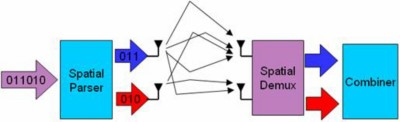Figure 9. MIMO System Uses Spatial Multiplexing to Increase Data Rate

As Figure 9 illustrates, spatial multiplexing requires multiple transmit and receive antennae. These antennae transmit and receive distinct data streams into the same physical channel.  As a result, engineers cannot evaluate the physical channel of a MIMO system in the same way that they can understand other communications channels.

Traditionally, communications channels have been represented by a vector approach such that the signal is known to have an instantaneous amplitude, frequency, and phase. However, a system with multiple transmitters using the portion of the spectrum is interpreted as mere noise if the traditional vector representation of the physical channel is used.

One way to view the physical channel is with a series of equations by which the received signal can be related as a function of the channel characteristics. For a single transmitter, single receiver communication channel (SISO), this relationship is represented rather easily with the equation in Figure 10: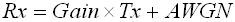Figure 10. Model of a Traditional Communications Channel 

In the Figure 10 equation, the received channel is represented as a function of antenna gain, the transmitted signal, and arbitrary white Gaussian noise (AWGN).

For MIMO systems, multiple transmitters use the same portion of frequency spectrum. In a traditional channel, this causes interference, and the receiver observes a signal that is a product of both transmitted signals. However, in MIMO systems, signal processing is used to reconstruct each of the transmitted streams and decode them individually. In general, this is implemented more easily when multiple receiver antennae are used. Fundamentally, this step of signal processing requires the receiver to estimate several basic characteristics of the transmitter and physical channel. These characteristics include gain, phase, and multipath effects. To generalize this relationship, each transmitted channel description is represented by a single variable, h. Thus, the system can be described with a channel model represented by the equation in Figure 11: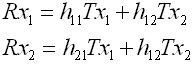Figure 11. MIMO Channel Equation 

In addition, this series of equations can be represented in a matrix form, as shown in Figure 12: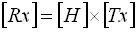Figure 12. Matrix Representation of a MIMO Channel 

Thus, for a 2x2 MIMO system, each receiver and transmitter antenna can be represented in the Figure 13 equation: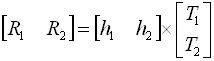Figure 13. Expanded Matrix Representation of a MIMO Channel 

As the series of equations above illustrate, the receiver is able to recover a signal that is a function of both the transmitted stream and the channel characteristics. Thus, for a MIMO system to operate, it is important to accurately estimate the phase and gain characteristics of each set of channel descriptors.

### Channel Estimation

As discussed earlier, the physical channel of a MIMO system can be modeled with the Figure 14 equation: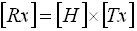Figure 14. Matrix Representation of a MIMO Channel 

Figure 14 shows that the received signal (Rx) is a function of the transmitted signal (Tx) and the channel description (H). To create a successful communications link, it is important to accurately characterize H for each transmit-receive pair. In addition, important characteristics include the amplitude and relative phase of the received signal. As a result, each spatial stream can be correlated to accurately estimate the original transmitted signal. This is illustrated in Figure 15: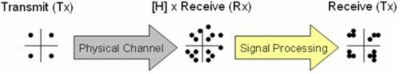Figure 15. Constellation Diagram for a MIMO Channel 

As Figure 15 illustrates, each of the transmitted streams can be mapped to ideal symbols on a constellation plot. When each spatial stream is generated into the same physical channel, each of these symbols appears to interfere with one another before being separated. Thus, before separation ([H] x [Rx]), simply viewing the physical channel with the same vector approach is insufficient. However, by estimating each channel independently and separating it into various spatial streams, engineers can map the seemingly garbled physical channel according to each transmitted stream.

Thus, the process of channel estimation is crucial to the implementation of a MIMO communications system. Typical implementations of MIMO transceivers must be designed such that baseband and RF subsystems are tightly synchronized between each antenna. In addition, while this is a challenge in system design, it is also an important challenge in MIMO test instrumentation. The following section explores various architectures that can be used to provide the best scenarios for instrument synchronization for MIMO test.

## Instrumentation Challenges of MIMO Test

Accurate testing of MIMO transceivers presents significant challenges to existing test instrumentation  architectures. New architectures require not only advanced signal processing algorithms to multiplex and demultiplex various spatial streams but also tight synchronization between each transmit and receive antenna. The following section addresses the challenges of synchronization and illustrates how a hybrid PXI/benchtop instrumentation approach can be used to provide baseband and RF synchronization.

### Baseband Synchronization

Synchronization of baseband generation and acquisition instrumentation is the first step to synchronizing RF signals. In a typical test configuration, baseband signals are generated and acquired with standard arbitrary waveform generators (AWGs) and high-speed digital oscilloscopes. On the generation side, the significance of baseband synchronization is illustrated with a conventional direct conversion modulator block diagram where t corresponds to the clock distribution delay between the two digital-to-analog converters (DACs). The discrete time samples of the in-phase (I) and quadrature (Q) waveforms given by I[n] and Q[n], respectively, are converted to analog waveforms I(t) and Q(t-t). This is shown in Figure 16: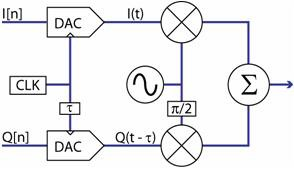Figure 16. Modulator with I/Q Skew Induced by Clock Delay

As Figure 16 illustrates, the local oscillator (LO) is mixed with each of the baseband I(t) and Q(t-t) signals to produce the RF output. Thus, phase skew between I[n] and Q[n] results in quadrature skew at the RF output.

With National Instruments PXI signal generators, the NI-TClk API can be used to synchronize multiple AWGs with a common 10 MHz system reference clock. In addition, these modules can be synchronized with a typical slot-to-slot skew of 100 ps with the following three VIs.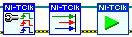Figure 17. Basic Functions of the NI-TClk Driver

In addition, baseband generators must also meet a bandwidth requirement. Because the IEEE 802.11n specification includes signals with a bandwidth of 40 MHz, multiple NI PXI-5422 AWGs (200 MS/s, 80 MHz bandwidth) can be synchronized to generate each of the baseband signals. Moreover, with high-bandwidth baseband signals, tight synchronization between instruments becomes critically essential.

Measuring the error vector magnitude (EVM) of a baseband signal is one way to characterize both the amplitude and phase accuracy of the generated signals. In addition, EVM measured from synchronized PXI-5422 modules is measured at -57 dB. This level is easily sufficient to meet the test requirements for 802.11n test systems.

This is illustrated in Figure 18, which shows the degradation in EVM as t increases from 100 to 1,000 ps for a 40 MHz bandwidth 802.11n signal.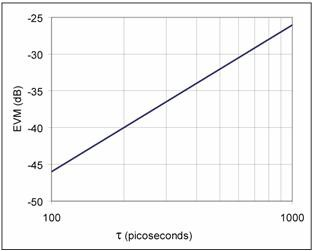Figure 18. EVM versus I/Q Skew for 40 MHz Bandwidth 802.11n Signal

Figure 18 shows that skew on the order of several hundred nanoseconds provides a hard-to-detect and significant EVM contribution into the measurement system. Skew on the order of milliseconds can render the measurement system useless for many testing applications.

The same skew requirements needed for baseband signal generation applies equally to baseband signal analysis. The multimodule synchronization capability is also built into the NI PXI-5124 digitizers and, therefore, provides an equally suitable solution for signal analysis of MIMO devices. These modules have 12-bit resolution and 200 MS/s sampling rate, and they also provide synchronization to within 20 ps. The EVM contribution from these modules was measured at -48 dB, which is well within the requirements for engineering grade test equipment. An added benefit of using both the PXI-5422 and PXI-5124 is that the signal generator and analyzer can be synchronized as well. This is useful for specific MIMO testing modes requiring simultaneous signal generation and signal analysis.

### Local Oscillator Synchronization

Tight baseband synchronization is only one step to ensure that each transmitter of a MIMO system is properly synchronized. As previously mentioned, a MIMO system requires that the phase between each transmitter antenna remain constant. When synchronizing baseband I and Q signals, skew should be minimal to prevent distortion of the RF signal. When synchronizing multiple RF signals, phase skew between each of the RF signals is tolerable but should be minimized as much as possible.

### Typical Test Architectures: The Hybrid Approach

One approach to ensure both baseband and RF synchronization is using a hybrid test architecture featuring both PXI and traditional instruments. With this architecture, customers can benefit both from the synchronization features available in PXI and the synchronization capabilities of benchtop RF instruments with a 10 MHz reference clock. A typical test architecture for a MIMO system is shown in Figure 19: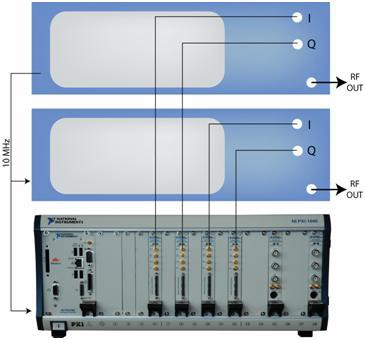Figure 19. Hybrid PXI/Traditional Instrument MIMO Test Architecture

As Figure 19 illustrates, a 2x2 MIMO configuration requires four channels of synchronized baseband I and Q signals. The baseband generation occurs in the PXI platform to take advantage of the synchronization capabilities of PXI. In addition, two benchtop vector signal generators are able to share a common 10 MHz reference clock to ensure synchronization of the RF signals.

### IEEE 802.11n Software Implementation

National Instruments recommends the Lyocom WLAN Test Suite software to provide a complete signal generation and signal analysis solution for the 802.11a/b/g/n standards. As described above, MIMO systems require significant signal processing to correlate each of the input streams from the RF antenna. Using digital signal processing (DSP) techniques in the NI LabVIEW programming environment, engineers can demultiplex and analyze each of the original transmitted streams.

The Lyocom WLAN Test Suite was developed for both stand-alone operation and automated testing environments. A menu-driven GUI combines intuitive hardware control with easy-to-navigate analysis controls and display options. The solution can also be seamlessly integrated into an automated testing environment with the extensive LabVIEW programming interface. The solution comes with more than 100 well-documented VIs providing a wide range of testing possibilities. Various examples and flow diagrams are also included to take the guesswork out of integration. A screenshot of the software suite is shown in Figure 20.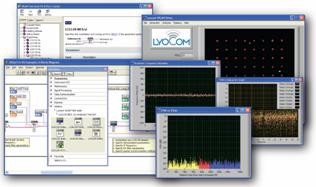Figure 20. WLAN Test Suite Software

### Conclusions

While MIMO communications systems and, specifically, the IEEE 802.11n standard present many new challenges for test engineers, these challenges can be met through the implementation of hybrid PXI/benchtop instrumentation. Fundamentally, this architecture is well-suited to address challenges of MIMO test systems including baseband synchronization, RF synchronization, and advanced signal processing routines.

### Resources

 E. Telatar, Capacity of Multiantenna Gaussian Channels. AT&T Bell Laboratories, Tech. Memo., June 1995.

 Martin and Christ  MIMO Channel Characterization and Joint Channel Estimation and Detection in Digital Communications. 2002.

 Kermoal, Schumacher, Pedersen, Mogensen, and Frank Frederiksen. A Stochastic MIMO Radio Channel Model With Experimental Validation. IEEE Journal on Selected Areas on Communications, August 2002.

 Gesbert, Shafi. Shiu, Smith, and Naguib. From Theory to Practice: An Overview of MIMO Space-Time Coded Wireless Systems. IEEE Journal on Selected Ares in Communications, April 2003.

 Badic, Rupp, and Weinrichter.  Adaptive Channel Matched Extended Alamouti Space-Time Code Exploiting Partial Feedback. ETRI Journal, October 2004.

 Taiwen Tang and Robert Heath. Space-Time Interference Cancellation in MIMO-OFDM Systems. IEEE Transactions on Vehicular Technology, 2005.